Test: Two Port Network - 2

# Test: Two Port Network - 2 - Electrical Engineering (EE)

Test Description

## 10 Questions MCQ Test Network Theory (Electric Circuits) - Test: Two Port Network - 2

Test: Two Port Network - 2 for Electrical Engineering (EE) 2023 is part of Network Theory (Electric Circuits) preparation. The Test: Two Port Network - 2 questions and answers have been prepared according to the Electrical Engineering (EE) exam syllabus.The Test: Two Port Network - 2 MCQs are made for Electrical Engineering (EE) 2023 Exam. Find important definitions, questions, notes, meanings, examples, exercises, MCQs and online tests for Test: Two Port Network - 2 below.
Solutions of Test: Two Port Network - 2 questions in English are available as part of our Network Theory (Electric Circuits) for Electrical Engineering (EE) & Test: Two Port Network - 2 solutions in Hindi for Network Theory (Electric Circuits) course. Download more important topics, notes, lectures and mock test series for Electrical Engineering (EE) Exam by signing up for free. Attempt Test: Two Port Network - 2 | 10 questions in 30 minutes | Mock test for Electrical Engineering (EE) preparation | Free important questions MCQ to study Network Theory (Electric Circuits) for Electrical Engineering (EE) Exam | Download free PDF with solutions
 1 Crore+ students have signed up on EduRev. Have you?
Test: Two Port Network - 2 - Question 1

### The two port matrix of an 1 : n ideal transfer is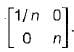It describes the transfer in terms of its

Detailed Solution for Test: Two Port Network - 2 - Question 1

An ideal transfer having turns ratio of 1 : n is shown below.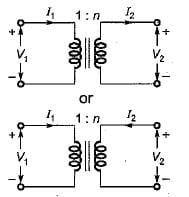Here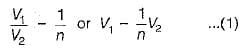Also,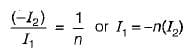or,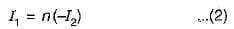Comparing equations (1) and (2) with standard transmission-parameter equations,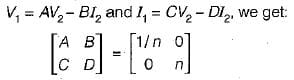Test: Two Port Network - 2 - Question 2

### Assertion (A): Simple resistors, inductors and capacitors are linear elements. Reason (R): The resistances, inductances and capacitances do not change with a change in applied voltage or the circuit current.

Test: Two Port Network - 2 - Question 3

### A two port network is not reciprocal if

Test: Two Port Network - 2 - Question 4

Assertion (A): Unilateral elements offer varying impedances with variations in flow of current while bilateral elements offer same impedance irrespective of flow of current.

Reason (R): If the magnitude of the current passing through an element is affected due to change in the polarity of the applied voltage, the element is called unilateral element while if the current magnitude remains the same even if the applied emf’s polarity is changed, it is called a bilateral element

Test: Two Port Network - 2 - Question 5

The  parameters Z11 a nd Z22 of the given T-network shown below are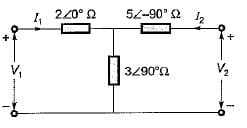Detailed Solution for Test: Two Port Network - 2 - Question 5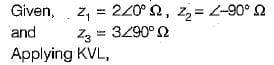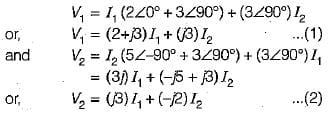Comparing equations (1) and (2) with the standard z-parameter equations;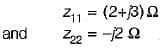Test: Two Port Network - 2 - Question 6

Match List-1 with List-11 and select the correct answer using the codes given below the lists: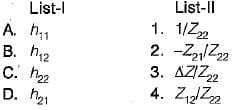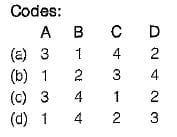Detailed Solution for Test: Two Port Network - 2 - Question 6

Z-parameter equations are: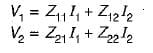and h parameter equations are: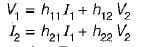Converting the Z-parameter equations into h-parameter equations, we have: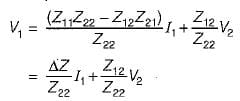and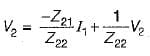Test: Two Port Network - 2 - Question 7

For the circuit given below, the value of the z12 parameter is ___________Detailed Solution for Test: Two Port Network - 2 - Question 7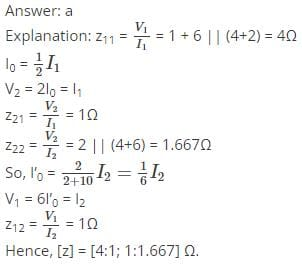Test: Two Port Network - 2 - Question 8

The value of Z21(s) in the circuit shown below is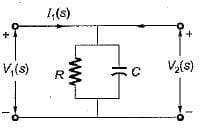Detailed Solution for Test: Two Port Network - 2 - Question 8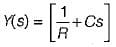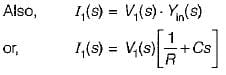As V1(s) = V2{s) therefore,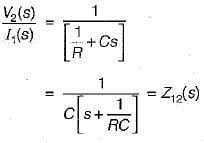Test: Two Port Network - 2 - Question 9

A two port network has zero initial conditions. At t = 0; an impulse voltage δ(t) is applied at the input producing a voltage e-2t + e-3t)  u(t) volts at the output. If the output voltage required is te-2t u(t) then, the input voltage will be

Detailed Solution for Test: Two Port Network - 2 - Question 9

Given:
Input voltge,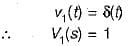and output,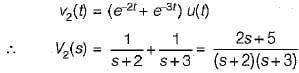Now, impulse response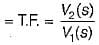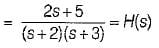Given, output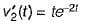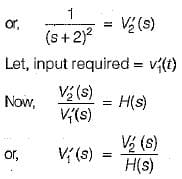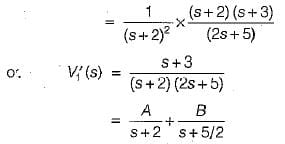Using partial fraction expansion, A = 1 and B = -0.5.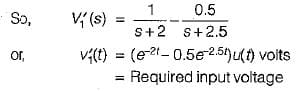Test: Two Port Network - 2 - Question 10

The Z-parameter of the two port network shown below is given by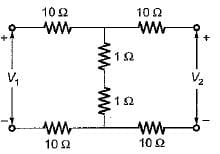Detailed Solution for Test: Two Port Network - 2 - Question 10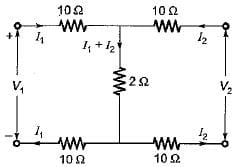Applying KVL in the two (oops,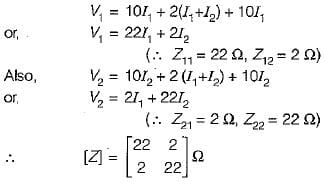## Network Theory (Electric Circuits)

45 videos|63 docs|61 tests
Information about Test: Two Port Network - 2 Page
In this test you can find the Exam questions for Test: Two Port Network - 2 solved & explained in the simplest way possible. Besides giving Questions and answers for Test: Two Port Network - 2, EduRev gives you an ample number of Online tests for practice

## Network Theory (Electric Circuits)

45 videos|63 docs|61 tests(Scan QR code)